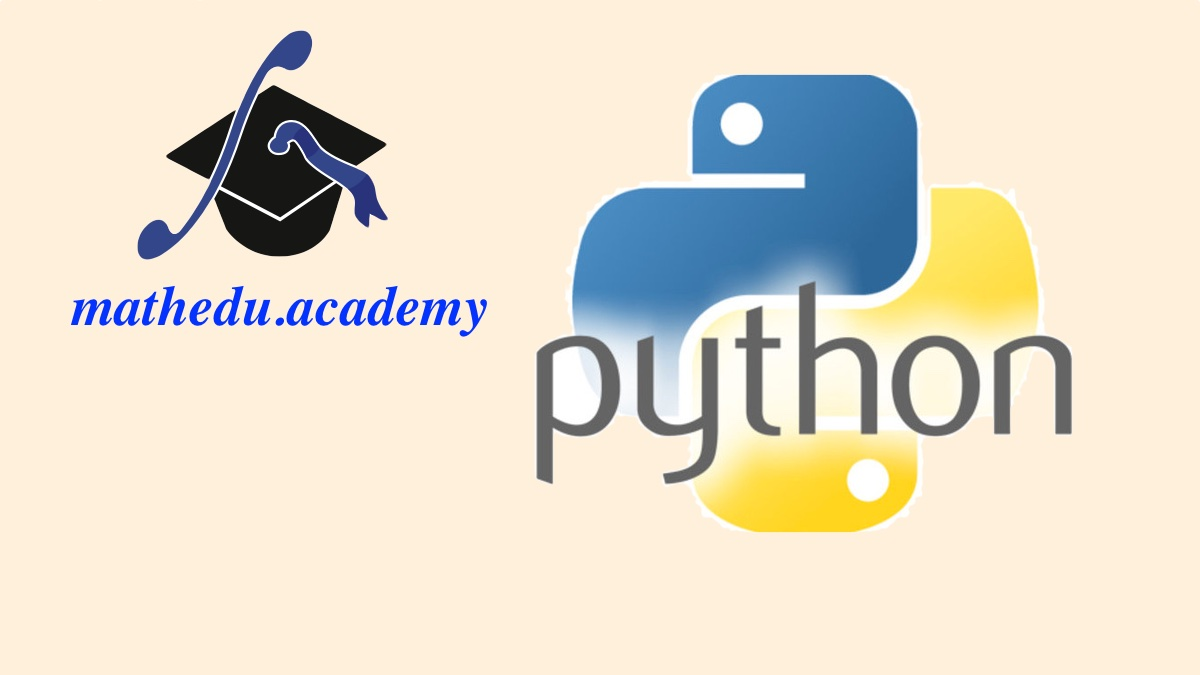# MatheduReengineering Mathematics# Scientific Computing with Python - the Basics

• Section 1: Preliminaries

### Lecture 0: HW&SW requirements

Before starting the core of the course, you will learn how to get Anaconda, the free distribtion of Python dedicated to scientific computing.

You will be invited to launch Anaconda navigator, and then the development environment Spyder, tollow profitabily the course.

### Lecture 1: Introduction

We start the course with a presentation of what will be taught in "Scientific Computing with Python - the Basics", and how it will be taught, with Python coding sessions for every video lecture.

• Section 2: Use Python as a Calculator

### Lecture 2: Arithmetics operations

Python executes arithmetic operations simply:

• the subtraction -
• the multiplication *
• the exponentiation **
• the division /

You will be invited to test these operations on Spyder console.

### Lecture 3: the Trigonometric Functions

The troigonometric functions are supported by 'numpy' library, that is included in Anaconda.

The consine cos, the sinus sin and the tangent tan are defined for angles in radians.

For the cotangent in radians, as well as the handling of angles in degrees (with conversion functions), a Python script is necessary, that is shown on the video and given in both downloadable .py and visualizable pdf formats.

### Lecture 4: Exponent related Functions

The 'numpy' package supports also the logaritmic functions, and their reciprocal the exponentiations.

More precisely, you will be invited to nanipulate:

• The natural logarithm log, and its reciprocal the exponential function exp
• The decimal logarithm log10, and its reciprocal the generalized power of 10, '10**x'
• Section 3: the Control Flow - start programming in Python

### Lecture 5: the Conditionals if elif else

You will learn how to program a conditional instruction, based on the example of defining a function that checks the parity of a number.

The script developed during the video is given as a downloadable .py file, and also as a readable pdf file.

### Lecture 6: The sequential loops for

In Python, the sequential loops for have an index in a list of any type, but we will only study the case of a list 'range' of consecutive integers.

A variant of that is the 'arange' one dimensional arrays of numpy, that has the advantage to cope well with the plotting function of MatPlotLib, studied in the next lecture.

• Section 4: Graphics with Numpy and MatPlotLib

### Lecture 7: Draw a sequence

You will learn here how to define and draw a sequence in Python, with the help of 'numpy' scientific computing package and 'matplotlib.pyplot' graphical package.

You will do it in a script, that is given in both downloadable .py file, and readable pdf file.

### Lecture 8: Draw a function

You will learn here how to define and draw a function of the real variable x in Python, with the help of 'numpy' scientific computing package and 'matplotlib.pyplot' graphical package.

You will do it in a script, that is given in both downloadable .py file, and readable pdf file.

• Section 5: Conclusion

### Lecture 9: Conclusion

Now, it's time to say good bye!

The master word is practice, and practice again.

And if you forcast to follow our series of courses of practical mathematics, now you are ready!